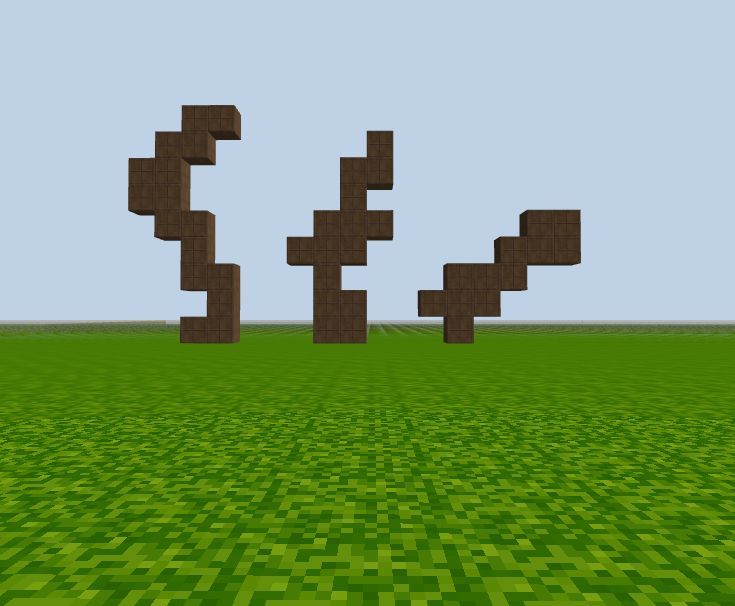# randInt() in CodeCraft

We use random integers a lot in CodeCraft. The function we defined `randInt(min, max)` is used to generate random integers.

### Example 1: Make an array of random integers:

``````let nums = [];

for (let i = 0; i < 5; i++) {
nums.push(randInt(1,20));
}

console.log(nums);
``````

Each time you run the above code, you should get a different set of numbers such as:

``````[11, 13, 8, 10, 10]
[16, 9, 16, 18, 8]
``````

### Example 2: build blocks at random locations with random material

Generating blocks at random locations would be easy with `randInt()`. For example, if you use `randInt(1, 20)` as the input value for the `x` coordinate, the block will show up at random `x` locations from 1 to 20.

To build blocks with random materials, let's use function `block_m(x, y, z, m)` where `m` is a number indicating the materials.

``````# random x, y, z, m
# 10 blocks with different materials at different locations
for (let i = 0; i < 10; i++) {
block_m(randInt(1, 20), randInt(1, 20), randInt(-20, -10), randInt(1, 88) );
}
``````

Each time you run the above code you will get a set of 10 different blocks at different locations.

### Example 3: Random columns

You can also generate columns of random materials with random heights. Let's use the function `column_m()`:

``````for (let i = 0; i < 10; i++) {
column_m(i, -10, randInt(1, 20), randInt(1, 16));
}
``````

Example 3 generated ten random colored columns with random heights.Run all the programs a few times and see what you get! Can you explain why some of these columns below have different color segments?### Example 4: random way to go

In this example we will grow a random plant. A random integer is used to control which way it grows into. 0: left, 1: right, 2: up.

``````// random direction
let x = 0;
let y = 1;

for (let i = 0; i < 20; i++) {
block(x, y, -30, "box_brown");

let direction = randInt(0, 3);

if (direction == 0) {
x -= 1;
}
else if (direction == 1) {
x += 1;
}
else {
y += 1;
}
}
``````Run the code three times, each time using a different initial x value: `-5, 0, 5`. We get 3 random "plants":